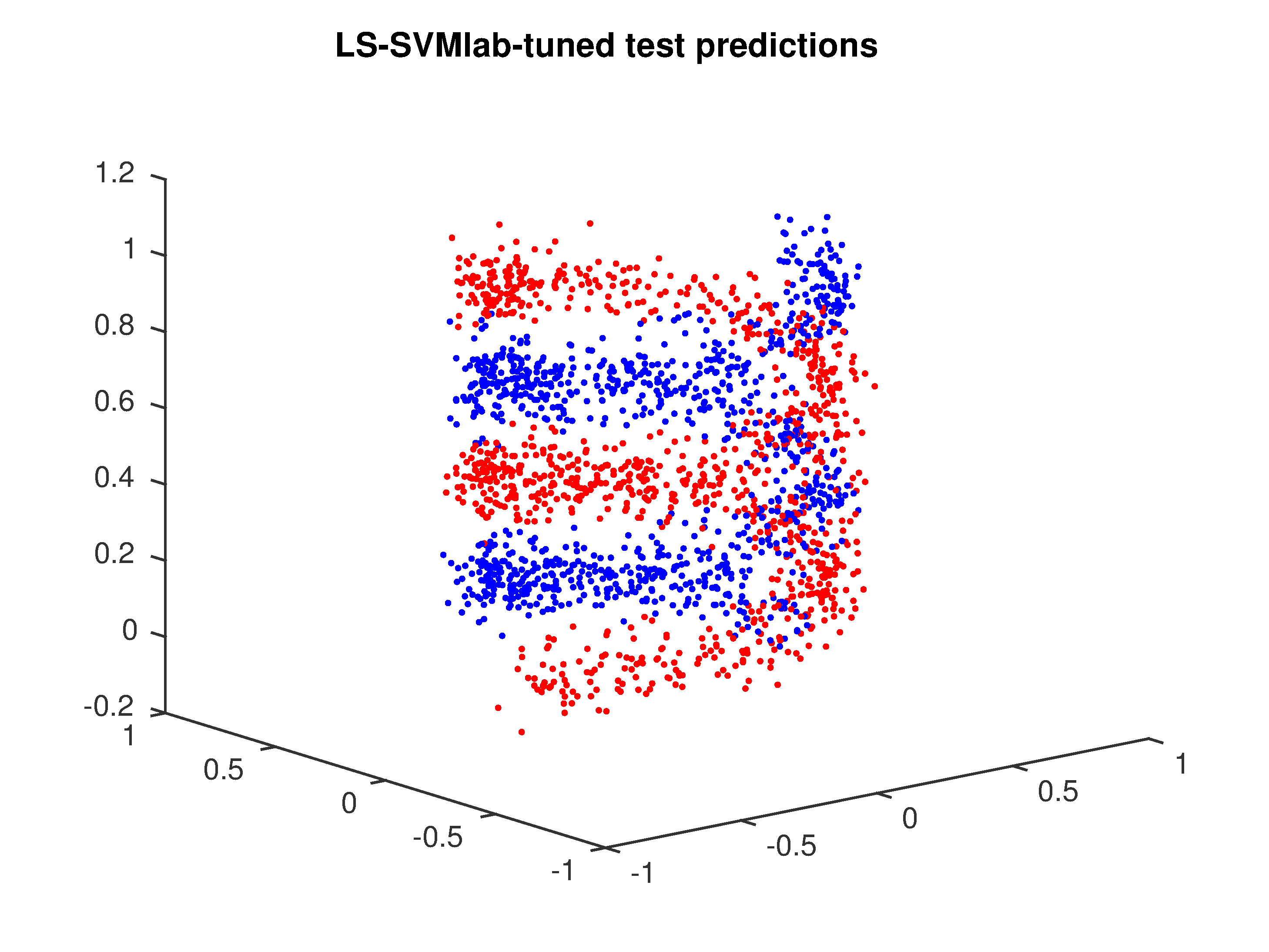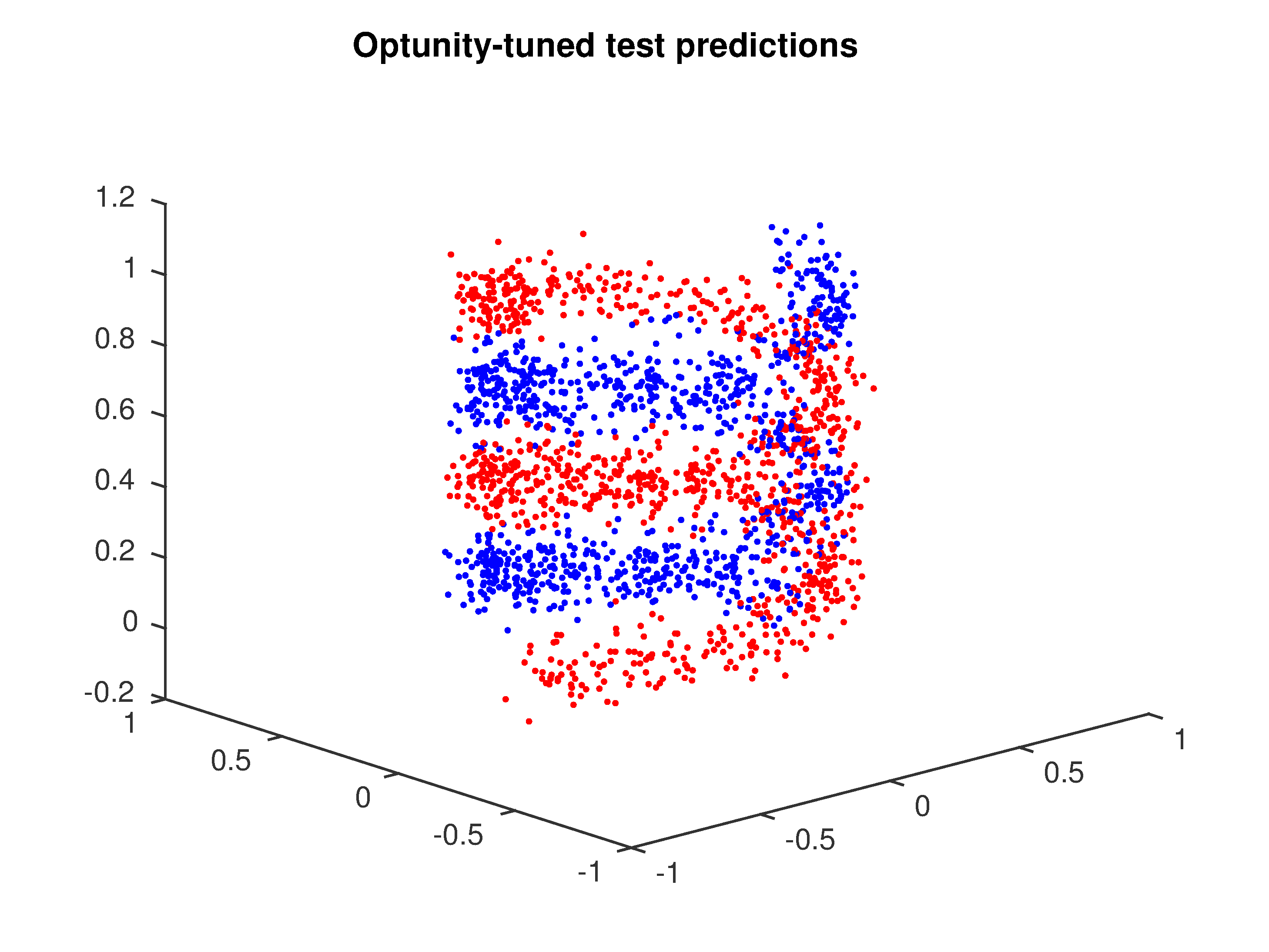# Least-squares SVM classification¶

You can find a MATLAB script for this example in <optunity>/wrappers/matlab/example_lssvmlab/demo_classification.m in your Optunity release.

In this example, we will perform nonlinear classification using LS-SVM with RBF kernel using the LS-SVMlab toolbox. In this case, we have to tune two hyperparameters: gam (regularization) and sig2 (kernel bandwidth). We will 10-fold cross-validation to test a pair of hyperparameters.

In this example, we will use optunity.minimize() to minimize misclassification rate.

Start off by defining the objective function, we will generate a double helix for classification:

r = 0.5;
h = 1;
helix = @(x, theta, n) [x + r*cos(theta + linspace(0, 4*pi, n)); ...
r*sin(theta + linspace(0, 4*pi, n)); linspace(0, h, n)]';

noise = 0.1;
ntr = 200;
pos = helix(0, 0, ntr);
neg = helix(0, pi, ntr);
X = [pos; neg] + noise*randn(2*ntr, 3);
Y = [ones(ntr, 1); -1*ones(ntr, 1)];


Now that we have training data, we can use LS-SVMlab and Optunity for tuning. In this example we use LS-SVMlab’s (verbose) functional API.

Tuning with LS-SVMlab’s built in procedure (in this case a combination of coupled simulated annealing and Nelder-Mead simplex):

[lssvm_gam, lssvm_sig2] = tunelssvm({X,Y,'c',[],[],'RBF_kernel'}, 'simplex',...
'leaveoneoutlssvm', {'misclass'});
[alpha_lssvm,b_lssvm] = trainlssvm({X,Y,type,lssvm_gam,lssvm_sig2,'RBF_kernel'});


To do the same with Optunity, we must first define an objective function, lets say demo_classification_misclass.m:

function [ misclass ] = demo_classification_misclass( x_train, y_train, x_test, y_test, pars )

% train model
[alpha,b] = trainlssvm({x_train,y_train,'classification',pars.gam,pars.sig2,'RBF_kernel'});

% predict test data
Yt = simlssvm({x_train,y_train,'classification',pars.gam,pars.sig2,'RBF_kernel','preprocess'},{alpha,b},x_test);

% compute misclassification rate
misclass = sum(Yt ~= y_test)/numel(Yt);
end


The objective function seems quite involved but it’s essentially training a model, predicting test data and evaluating. The code to perform the actual tuning (we use 10-fold cross-validation):

obj_fun = optunity.cross_validate(@demo_classification_misclass, X, 'y', Y, 'num_folds', 10);
opt_pars = optunity.minimize(obj_fun, 100, 'gam', [1, 100], 'sig2', [0.01, 2]);
[alpha_optunity,b_optunity] = trainlssvm({X,Y,type,opt_pars.gam,opt_pars.sig2,'RBF_kernel'});


Now we have two LS-SVM models, one tuned with LS-SVM’s own algorithms and one using Optunity. For this particular problem, their performance is close to equivalent. The figures below shows test set predictions for both models.Test set predictions of LS-SVM after tuning with LS-SVMlab.Test set predictions of LS-SVM after tuning with Optunity.